×

Search anything:

# Evaluation metrics for object detection and segmentation

#### Machine Learning (ML)Indian Technical Authorship Contest starts on 1st July 2023. Stay tuned.

In this article, we will go through the Evaluation metrics for Object Detection and Segmentation that is Image segmentation, semantic segmentation and instance segmentation in depth.

#### 6. mAP (mean Average Precision)

Following table summarizes the concept:

Evaluation MetricCalculationUsed for
Pixel AccuracyPixel Accuracy = Number of correct pixels / Number of all pixelsNot recommended to use. Image segmentation, semantic segmentation and instance segmentation
True positive (TP), False positive (FP), False negative (FN) and True negative (TN)Number of right match and mis-match to wrong match and mis-matchMainly for Object Detection
IoU (Intersection over union)Area of overlap between the predicted bounding box and the ground-truthObject Detection
Precision and RecallPercent of truly predicted objectsMainly for Object Detection; Limited use in Image segmentation, semantic segmentation and instance segmentation
Average Precision (AP), mAP (mean Average Precision)Area under the Precision-Recall curveImage segmentation, semantic segmentation and instance segmentation

Dive deeper into the concept.

## 1. Difference between image classification, object detection and image segmentation:

• image classification : it is when you have single object in an image and want to classify it. like it is a dog or a cat?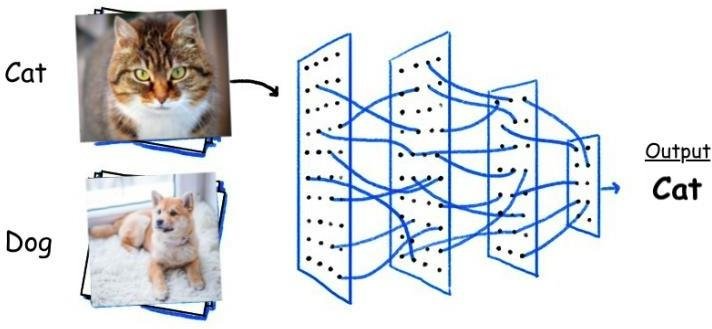• object detection :it is when you have multiple objects in your image and want to detect them with their location or their rectangular boundary boxes.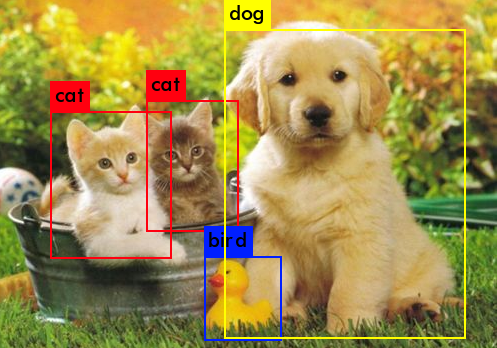this photo may explain the difference more obviously.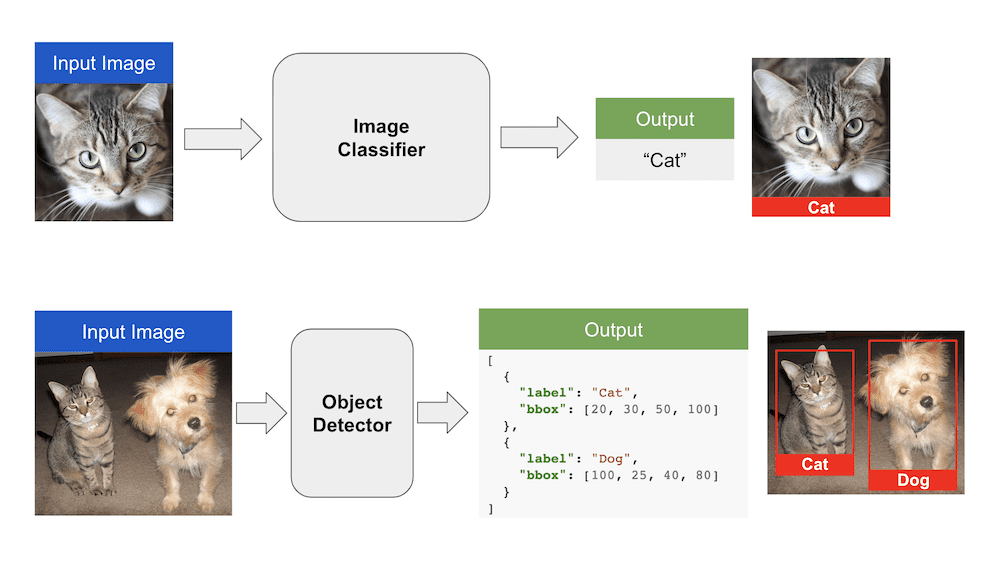• image segmentation: it is a technique than trying to detect object by classifying each single pixel to which object it belong. Image segmentation may be classified to many classes like semantic segmentation and instance segmentation.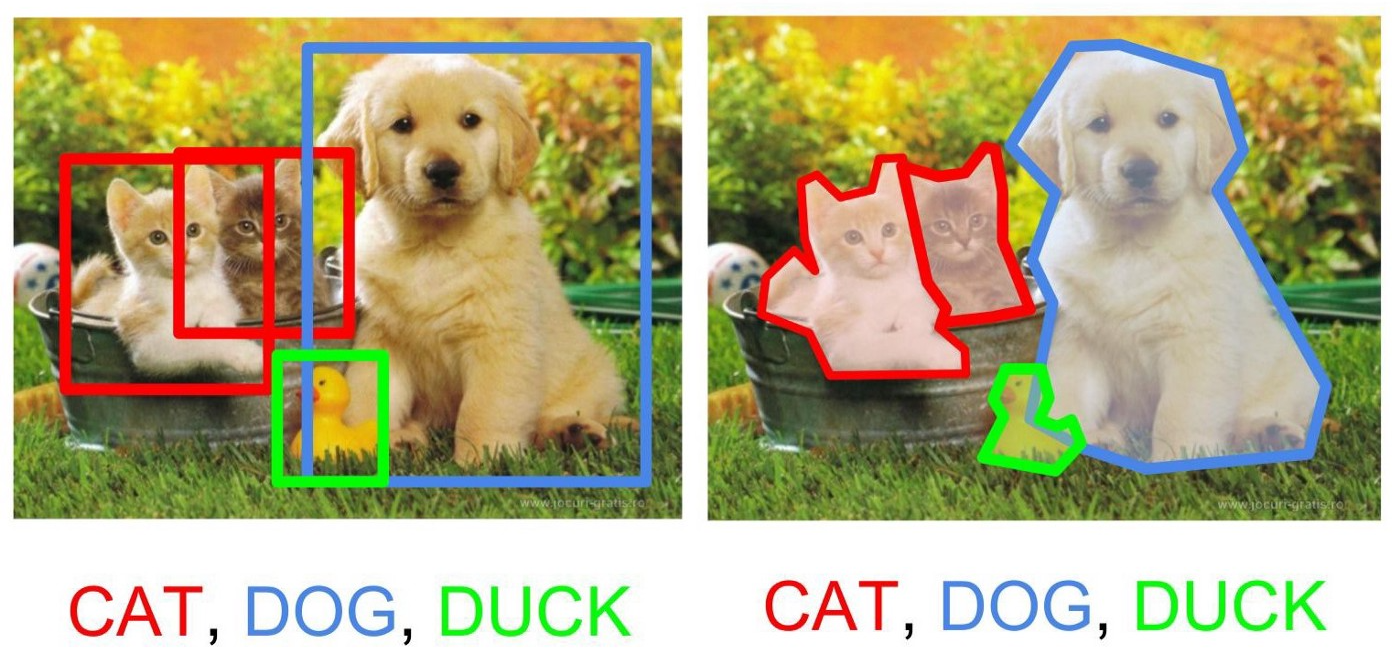the total difference between them in this photo.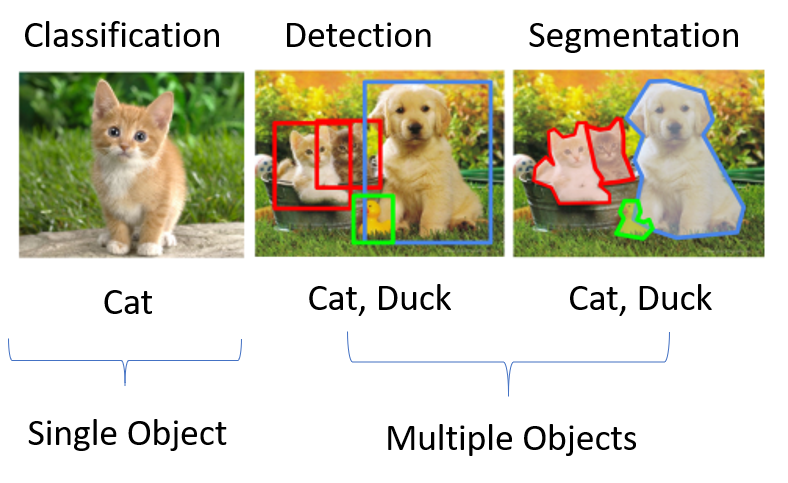After we trained our deep neural network model we need to evaluate if it work well or not, So we have many evaluation metrics for this task such as accuracy, precision, recall, sensitivity, specificity, AUC, mAP and others.

We don't have a single perfect metric which can work for each task, So let's talk about some of them which may work for object detection and image segmentation.

## 2. Pixel Accuracy

It is the easiest metric to explain as it mean percentage of pixels in your image that your model classified correctly. but as we know that accuracy not always a good metric.

Pixel Accuracy = No. of correct pixels/No. of all pixels

## 3. True positive to negatives

Now we will talk about very important concepts in evaluation metrics which are :

1. True positive (TP) : A correct detection of ground-truth bounding box.(the model detect an object that is already present).
2. False positive (FP) : An incorrect detection of non-existing bounding box or object. (the model detect an object that is not present in fact).
3. False negative (FN) : The undetected ground-truth bounding box. (the model unable to detect an object that is already present).
4. True negative (TN): The undetected non-existing bounding box or object. it is infinite number and we don't consider it in our calculation.

Now we can differentiate between them but there is a very critical concept in there definitions "the word CORRECT" . when we can said these detected boundary box is correct or incorrect. This will moving us to the next metric.

## 4. IoU (Intersection over union) :

The intersection over union or what is said as the Jaccard Index is the area of overlap between the predicted bounding box and the ground-truth divided by the area of union between the predicted bounding box and the ground truth bounding box.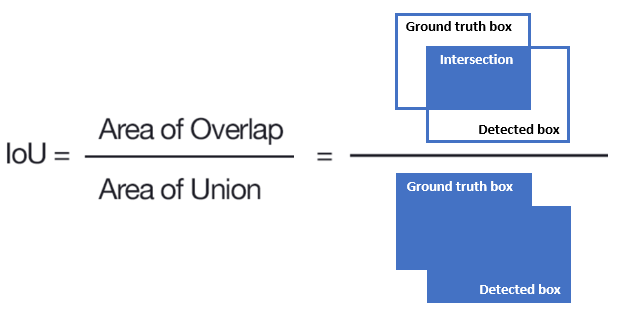Now we can use a threshold for this IOU values to determination the correction of detecting bounding box. For example let's say we choose the threshold = 0.5, So each detected bounding box with IOU above 0.5 consider "correct" and if the detected bounding box with IOU less than 0.5 consider "incorrect"

## 5. Precision and Recall :

Let us introduce a new concepts that depend on the previously illustrated ones thats called:

• Precision : is the percent of truly predicted objects to total positive predicted objected. (for total objects that your model predict as positive, how many of them are true).
Precision = (TP)/(TP+FP)
• Recall : IS the percent of truly predicted objects to total positive ground-truth objected. ( for total ground-truth objects that present how many your model can predict truly ).
Recall = (TP)/(TP+FN) or (TP)/all ground-truth objects.

## 6. mAP (mean Average Precision)

Let's consider our test dataset is consist of 4 images and our model get their all predicted bounding boxes with corresponding confidence level of each box.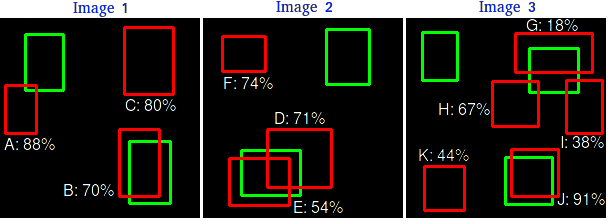Then descending sort all predictions according confidence level and calculate precision and recall as we go through.

prediction confidence TP or FP Precision Recall
J 91 TP 1 0.143
A 88 FP 0.5 0.143
C 80 FP 0.333 0.143
F 74 FP 0.25 0.143
D 71 FP 0.20 0.143
B 70 TP 0.333 0.286
H 67 FP 0.286 0.286
E 54 TP 0.375 0.426
k 44 FP 0.333 0.426
I 38 FP 0.3 0.426
G 18 TP 0.364 0.571

then plot precision versus recall: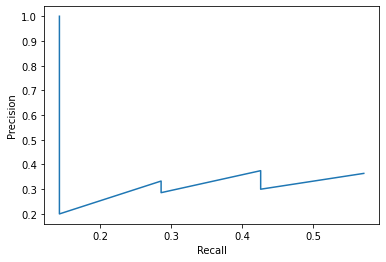Then calculate area under the Precision-Recall curve and generally it is the AP.
But we often try to smooth that zigzag shape to reduce the impact of the “wiggles” in the precision/recall curve, caused by small variations in the ranking of examples by replacing each precision value at each recall point with the maximum precision value to the right of that recall point.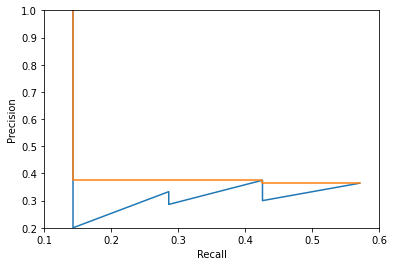So, Average Precision (AP) = AUC for precision-recall curve.
This was only for one class and we need to calculate AP for other classes by the same way then find the mean for them.

mAP (mean average precision)= Sum of AP for each class / n .
where n is number of classes.
Nowadays thanks to multiple AI frameworks you don't need to calculate these metrics from scratch. tensorflow and keras provide all of these metrics in just few lines of code:

For mAP use:

``````tfr.keras.metrics.MeanAveragePrecisionMetric(
name=None, topn=None, dtype=None, ragged=False, **kwargs)
``````

For Recall use:

``````tf.keras.metrics.Recall(
thresholds=None, top_k=None, class_id=None, name=None, dtype=None
)
``````

For Precision use:

``````tf.keras.metrics.Precision(
thresholds=None, top_k=None, class_id=None, name=None, dtype=None
)
``````

With this article at OpenGenus, you must have the complete idea of Evaluation metrics for object detection and Image segmentation including semantic segmentation and instance segmentation.

Finally, I want to thank you for reading.#### Ahmed Mandour

Ahmed Mandour is working at National Food Safety Authority and was studied at Mansoura University.